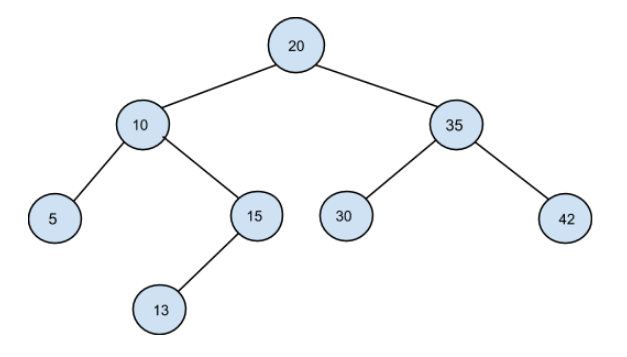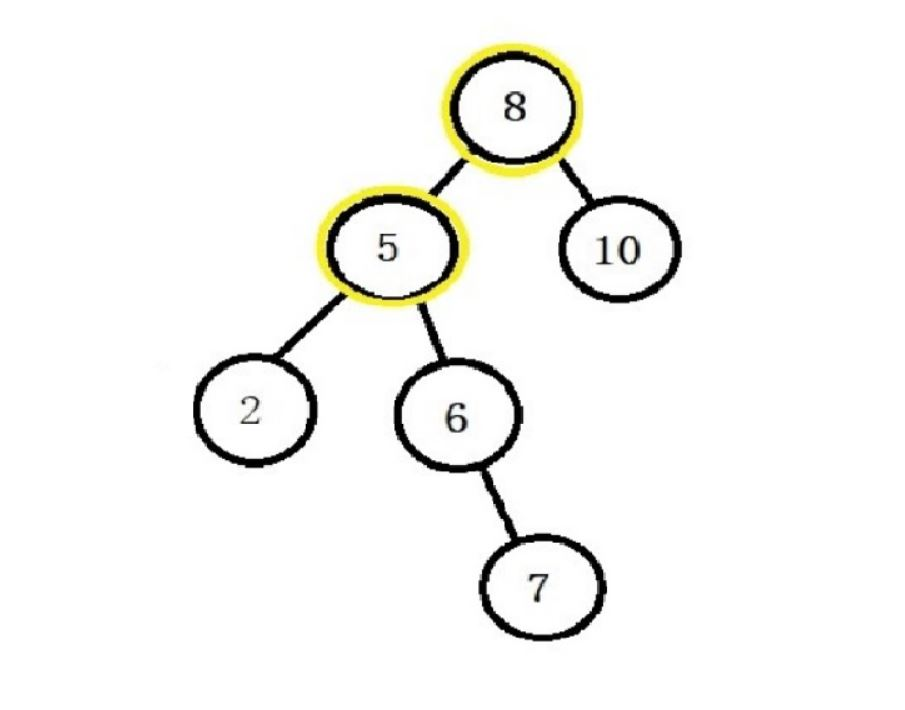BST Notes
BST Delete
Total Number of BSTs using array elements as the root node
Sorted Linked List to Balanced BST
Find K-th smallest Element in BST
Predecessor And Successor In BST
Binary Tree To BST
Pair Sum in BST
Convert Bst To The Greater Sum Tree
Two Sum in a BST
Validate BST1

# Pair Sum in BST.

Difficulty: EASY
Avg. time to solve
15 min
Success Rate
85%

Problem Statement
Suggest Edit

#### A binary search tree (BST) is a binary tree data structure which has the following properties.

``````• The left subtree of a node contains only nodes with data less than the node’s data.
• The right subtree of a node contains only nodes with data greater than the node’s data.
• Both the left and right subtrees must also be binary search trees.
``````
##### Note:
``````1. All the elements of the Binary Search Tree are unique.
2. You can’t use the same node value/element of BST twice.
``````
##### Input Format:
``````The first line of input contains an integer ‘T’, which denotes the number of test cases. Then each test case  follows.

The first line of every test case contains elements of Binary Search Tree in the level order form. The input consists of values of nodes separated by a single space in a single line. In case, a node is null, we take -1 in its place.

The second line of every test case contains a single integer ‘K’ which denotes the target value.
``````
##### For example:
``````The input for the tree depicted in the below image would be :
````````````20 10 35 5 15 30 42 -1 13 -1 -1 -1 -1 -1 -1 -1
``````
##### Explanation :
``````Level 1 :
The root node of the tree is 1

Level 2 :
Left child of 1 = 2
Right child of 1 = 3

Level 3 :
Left child of 2 = 4
Right child of 2 = null (-1)
Left child of 3 = 5
Right child of 3 = 6

Level 4 :
Left child of 4 = null (-1)
Right child of 4 = 7
Left child of 5 = null (-1)
Right child of 5 = null (-1)
Left child of 6 = null (-1)
Right child of 6 = null (-1)

Level 5 :
Left child of 7 = null (-1)
Right child of 7 = null (-1)

The first not-null node (of the previous level) is treated as the parent of the first two nodes of the current level. The second not-null node (of the previous level) is treated as the parent node for the next two nodes of the current level and so on.

The input ends when all nodes at the last level are null (-1).
``````

#### Note:

``````The above format was just to provide clarity on how the input is formed for a given tree.

The sequence will be put together in a single line separated by a single space. Hence, for the above-depicted tree, the input will be given as:

1 2 3 4 -1 5 6 -1 7 -1 -1 -1 -1 -1 -1
``````
##### Output Format:
``````For each test case, print a single line containing “true” or “false”.
``````

#### Note:

``````You don’t need to print the output, it has already been taken care of. Just implement the given function.
``````

#### Constraints:

``````1 <= T <= 5
1 <= N <= 10 ^ 3
0 <= DATA <= 10 ^ 9
1 <= K <= 10 ^ 9

Where ‘DATA’ denotes the value of each node in the given tree and ‘N’ denotes the number of nodes in BST.

Time limit: 1 sec
``````
##### Sample Input 1:
``````2
8 5 10 2 6 -1 -1 -1 -1 -1 7 -1 -1
13
8 5 10 2 6 -1 -1 -1 -1 -1 7 -1 -1
19
``````
##### Sample Output 1:
``````true
false
``````
##### Explanation for sample input 1:
``````For the first test case, the nodes with values 8 and 5 as shown in the below diagram, gives sum equal to the given target 13. Therefore, the output will be “true” i.e it is possible to find a pair in the given BST having sum equal to ‘K’.

For the second test case, there are no two elements in the given BST such that their sum is equal to the given target ‘K’ = 19.
``````##### Sample Input 2 :
``````2
20 16 -1 12 -1 8 -1 4 -1 -1 -1
12
15 10 20 8 12 16 25 -1 -1 -1 -1 -1 -1 -1 -1
57
``````
##### Sample Output 2:
``````true
false
``````Console